# Example Problem Solution

[Page 93]

This example demonstrates the transformation of a linear programming model into standard form, sensitivity analysis, computer solution, and shadow prices.

#### Problem Statement

The Xecko Tool Company is considering bidding on a job for two airplane wing parts . Each wing part must be processed through three manufacturing stagesstamping, drilling, and finishingfor which the company has limited available hours. The linear programming model to determine how many of part 1 ( x 1 ) and part 2 ( x 2 ) the company should produce in order to maximize its profit is as follows :

maximize Z = \$650 x 1 + 910 x 2

subject to

4 x 1 + 7.5 x 2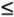105 (stamping, hr.)

6.2 x 1 + 4.9 x 290 (drilling, hr.)

9.1 x 1 + 4.1 x 2110 (finishing, hr.)

x 1 , x 2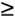1. Solve the model graphically.

2. Indicate how much slack resource is available at the optimal solution point.

3. Determine the sensitivity ranges for the profit for wing part 1 and the stamping hours available.

4. Solve this model by using Excel.

#### Solution

1.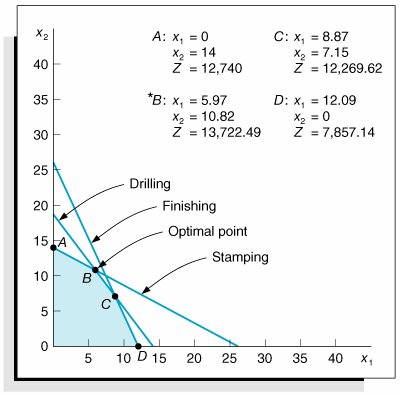2. The slack at point B , where x 1 = 5.97 and x 2 = 10.82, is computed as follows:

4(5.97) + 7.5(10.82) + s 1 = 105 (stamping, hr.)

s 1 = 0 hr.

6.2(5.97) + 4.9(10.82) + s 2 = 90 (drilling, hr.)

s 2 = 0 hr.

9.1(5.97) + 4.1(10.82) + s 3 = 110 (finishing, hr.)

s 3 = 11.35 hr.

[Page 94]
3. The sensitivity range for the profit for part 1 is determined by observing the graph of the model and computing how much the slope of the objective function must increase to make the optimal point move from B to C . This is the upper limit of the range and is determined by computing the value of c 1 that will make the slope of the objective function equal with the slope of the constraint line for drilling, 6.2 x 1 + 4.9 x 2 = 90: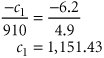The lower limit is determined by computing the value of c 1 that will equate the slope of the objective function with the slope of the constraint line for stamping, 4 x 1 + 7.5 x 2 = 105: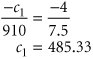Summarizing,

485.33c 11,151.43

The upper limit of the range for stamping hours is determined by first computing the value for q 1 that would move the solution point from B to where the drilling constraint intersects with the x 2 axis, where x 1 = 0 and x 2 = 18.37: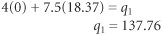The lower limit of the sensitivity range occurs where the optimal point B moves to C , where x 1 = 8.87 and x 2 = 7.15: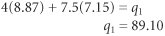Summarizing, 89.10q 1137.76.Introduction to Management Science (10th Edition)
ISBN: 0136064361
EAN: 2147483647
Year: 2006
Pages: 358

Similar book on Amazon

flylib.com © 2008-2017.
If you may any questions please contact us: flylib@qtcs.net